WO2019036927A1 - Stride frequency based foot step counting method, apparatus and device - Google Patents

Stride frequency based foot step counting method, apparatus and device Download PDF

Info

Publication number
WO2019036927A1
WO2019036927A1 PCT/CN2017/098591 CN2017098591W WO2019036927A1 WO 2019036927 A1 WO2019036927 A1 WO 2019036927A1 CN 2017098591 W CN2017098591 W CN 2017098591W WO 2019036927 A1 WO2019036927 A1 WO 2019036927A1
Authority
WO
WIPO (PCT)
Prior art keywords
value
feature
time interval
signal
set
Prior art date
Application number
PCT/CN2017/098591
Other languages
French (fr)
Chinese (zh)
Inventor

Original Assignee

Priority date (The priority date is an assumption and is not a legal conclusion. Google has not performed a legal analysis and makes no representation as to the accuracy of the date listed.)
Filing date
Publication date
Application filed by 华为技术有限公司 filed Critical 华为技术有限公司
Priority to PCT/CN2017/098591 priority Critical patent/WO2019036927A1/en
Publication of WO2019036927A1 publication Critical patent/WO2019036927A1/en

• 230000001133 acceleration Effects 0 abstract claims description 69
• 230000003044 adaptive Effects 0 description 1
• 238000004364 calculation methods Methods 0 claims description 8
• 230000001721 combination Effects 0 description 1
• 238000004891 communication Methods 0 description 2
• 238000004590 computer program Methods 0 claims description 14
• 230000000875 corresponding Effects 0 claims description 49
• 230000001808 coupling Effects 0 description 3
• 238000010168 coupling process Methods 0 description 3
• 238000005859 coupling reaction Methods 0 description 3
• 238000005265 energy consumption Methods 0 description 1
• 230000036545 exercise Effects 0 abstract claims description 18
• 238000000605 extraction Methods 0 description 2
• 239000000284 extracts Substances 0 claims description 11
• 238000001914 filtration Methods 0 claims 2
• 238000009963 fulling Methods 0 claims description 19
• 230000001965 increased Effects 0 description 2
• 239000003138 indicator Substances 0 description 1
• 239000011133 lead Substances 0 description 1
• 239000002609 media Substances 0 claims description 6
• 230000015654 memory Effects 0 claims description 10
• 238000000034 methods Methods 0 description 4
• 239000000203 mixtures Substances 0 description 2
• 230000003287 optical Effects 0 description 1
• 230000036961 partial Effects 0 description 1
• 230000000737 periodic Effects 0 description 1
• 230000037081 physical activity Effects 0 description 1
• 230000000704 physical effects Effects 0 description 1
• 239000011295 pitch Substances 0 claims 1
• 239000000047 products Substances 0 description 1
• 238000010010 raising Methods 0 description 1
• 230000000717 retained Effects 0 description 1
• 230000000630 rising Effects 0 claims description 8
• 238000005070 sampling Methods 0 claims description 9
• 230000011218 segmentation Effects 0 description 1
• 230000003068 static Effects 0 description 3
• 230000001429 stepping Effects 0 description 2
• 238000003860 storage Methods 0 claims description 6
• 230000001131 transforming Effects 0 claims description 4

Images

•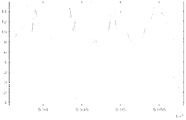•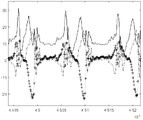•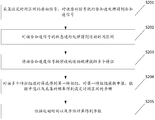•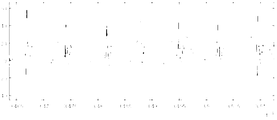•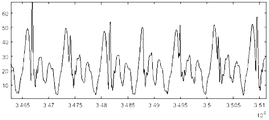••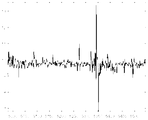••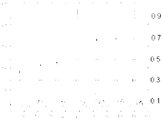•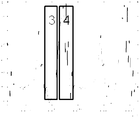••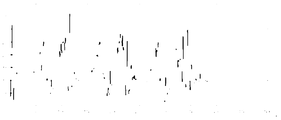•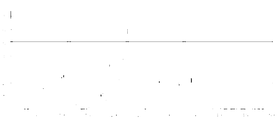•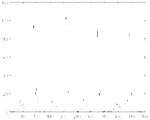•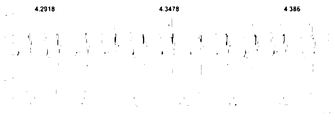•••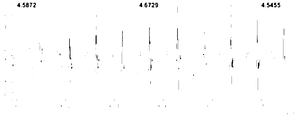•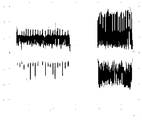•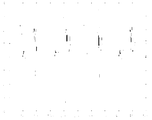••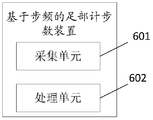•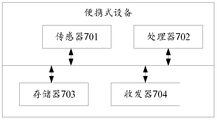Classifications

• GPHYSICS
• G01MEASURING; TESTING
• G01CMEASURING DISTANCES, LEVELS OR BEARINGS; SURVEYING; NAVIGATION; GYROSCOPIC INSTRUMENTS; PHOTOGRAMMETRY OR VIDEOGRAMMETRY
• G01C22/00Measuring distance traversed on the ground by vehicles, persons, animals or other moving solid bodies, e.g. using odometers, using pedometers

Abstract

The embodiment of the present application discloses a step frequency-based foot counting method, apparatus and system, the method comprising the steps of: collecting an original signal of a set time interval, performing an acceleration processing on the original signal to obtain a combination Accelerating a signal; performing state processing on the combined acceleration signal to obtain a motion time interval, extracting a plurality of feature groups according to a preset motion law; and screening the plurality of feature groups to obtain a first feature group, Extracting a median median value for the first feature set, obtaining a step frequency of the set time interval according to the median value and the collected frequency; calculating a step number according to the exercise time interval and the step frequency. This application has the advantage of achieving a foot step.

Description

Step frequency based foot counting method, device and device Technical field

The present application relates to the field of communications, and in particular, to a method, device and device for step counting based on a step frequency.

Background technique

Under the guidance of the National Fitness National Strategy, running sports are well received by the masses, and there are huge market opportunities for wearable sports equipment. Fitness record is the basic exercise and fitness function in wearable sports equipment. It mainly realizes: step counting, distance recording, motion time recording, energy consumption calculation, etc. Most users have this function. One of the most intuitive sports indicators in fitness records is that it not only allows users to directly assess their own physical activity, but also integrates with relevant social apps and becomes an important part of sports socialization.

The existing wearable exercise device is based on the step of the wrist and the waist wearing part. If the wearing part of the user is the foot, the step can not be realized, so the existing technical solution cannot realize the step counting for the foot.

Summary of the invention

The technical problem to be solved by the embodiments of the present application is to provide a step counting method based on the step frequency, which can solve the problem that the foot counting cannot be realized in the prior art.

In a first aspect, a step frequency based foot counting method is provided, the method comprising the steps of: acquiring an original signal of a set time interval, performing an acceleration processing on the original signal to obtain a combined acceleration signal; The acceleration signal is subjected to state processing to obtain a motion time interval, and the acceleration signal is extracted according to a preset motion law to extract a plurality of feature groups; and the plurality of feature groups are filtered to obtain a first feature group, and the first feature group is obtained Extracting a median value, obtaining a step frequency of the set time interval according to the median value and the collected frequency; calculating the number of steps according to the motion time interval and the step frequency.

The above technical solution can obtain a plurality of feature groups by processing the foot acceleration information, extract a median value of the first feature group from the plurality of feature groups, obtain a step frequency, and obtain a step number by using a step frequency calculation, thereby implementing the step based The frequency is implemented.

In an alternative of the first aspect, the step number = the set time interval length * the exercise time ratio / the step frequency;

Or the number of steps = length of the exercise time interval / step frequency.

In another optional aspect of the first aspect, the performing the state processing on the combined acceleration signal to obtain a motion time interval includes:

The acceleration signal is divided into sub-windows of a set interval, and the maximum value, the minimum value, the average value, and the number of extreme points of the signals in each sub-window are extracted, according to the maximum value, the minimum value, the average value, and the pole The range of the number of value points determines the corresponding state of each sub-window, and the sum of the time intervals of the sub-windows corresponding to the walking state and the running state is the motion time interval.

In still another optional aspect of the first aspect, the summing acceleration signal extracts a plurality of feature groups according to a preset motion rule, including:

Performing a plurality of differential processing on the combined acceleration signal to obtain a signal after multiple differential processing, and setting n adjacent values in the plurality of differentially processed signals to a small window, and finding n small windows for each small window The sum of the values, transforming the sum of the n values of each small window into a second differential processing and signal;

Finding a maximum amplitude M max of the multiple differential processing and signal; configuring a plurality of threshold lines for the multiple differential processing and signal, and a difference between adjacent two threshold lines is M max *C%, wherein C is a fixed value;

Obtaining a number m of intersection points of each threshold line and a plurality of differential processing and rising edges of the signal, and a value corresponding to the X axis of each intersection point, and using the value of the m and each intersection point corresponding to the X axis as a feature corresponding to the threshold line Groups, the feature groups corresponding to all threshold lines are the plurality of feature groups.

In a next alternative of the first aspect, the screening the plurality of feature groups to obtain a first feature set, extracting a median value for the first feature set, according to the median value and the frequency of acquisition Obtaining the step frequency of the set time interval, including:

Extracting p feature groups of the plurality of feature groups with m in a set range, and calculating a normalized standard deviation value of values of X axes of each of the p feature groups;

Calculating a difference value of the number of intersections of two adjacent feature groups in the p feature groups to obtain a first difference group, and calculating a normalization standard of values of X axes of adjacent two feature groups in the p feature groups The difference value of the difference is obtained by the second difference group, and the ith value of the first difference group is extracted and the ith value of the second difference group is weighted to obtain a result of the plurality of weighting operations, and the plurality of weighting operations are performed. The result is a first j-weighted operation result that is greater than the weighted threshold from the front to the back, and the forward feature set of the j-th weighted operation result is determined as the first feature set; the i, j are integers greater than or equal to 1;

A median value of the value of the X-axis of the first feature set, a step frequency = a median median value/sampling frequency is extracted.

In a second aspect, a step frequency based foot counting device is provided, the device comprising:

An acquisition unit, configured to acquire an original signal of a set time interval; a processing unit, configured to perform an acceleration process on the original signal to obtain a combined acceleration signal; and perform state processing on the combined acceleration signal to obtain a motion time interval, where Combining the acceleration signal to extract a plurality of feature groups according to a preset motion rule; screening the plurality of feature groups to obtain a first feature group, extracting a median value from the first feature group, according to the median value and collecting The frequency is obtained as a step frequency of the set time interval; the number of steps is calculated according to the motion time interval and the step frequency.

In a third aspect, a portable device is provided, the device comprising: a sensor, a processor, a memory, and a transceiver, the processor being coupled to the sensor, the memory, and the transceiver, wherein the sensor, And a processor configured to perform acceleration processing on the original signal to obtain a combined acceleration signal; and performing state processing on the combined acceleration signal to obtain a motion time interval, where the combination is The acceleration signal extracts a plurality of feature groups according to a preset motion rule; screening the plurality of feature groups to obtain a first feature group, extracting a median value from the first feature group, according to the median value and the collected The frequency obtains a step frequency of the set time interval; and the step number is calculated according to the motion time interval and the step frequency.

In a fourth aspect, a computer readable storage medium is provided, characterized in that it stores a computer program for electronic data exchange, wherein the computer program causes the computer to perform the method provided by the first aspect.

In a fifth aspect, a computer program product is provided, comprising: a non-transitory computer readable storage medium storing a computer program, the computer program being operative to cause a computer to perform the first aspect method.

DRAWINGS

In order to more clearly illustrate the embodiments of the present application or the technical solutions in the prior art, the drawings to be used in the embodiments will be briefly described below. Obviously, the drawings in the following description are only some of the present application. Embodiment, Other drawings may also be obtained from those of ordinary skill in the art in light of the inventive work.

Figure 1a is a waveform diagram of a hand walk signal.

Figure 1b is a waveform diagram of an ankle walking signal.

FIG. 2 is a schematic flowchart diagram of a step frequency based foot counting method according to an embodiment of the present application.

FIG. 2a is an original signal for collecting an ankle of a set time interval according to an embodiment of the present application.

FIG. 2b is a schematic diagram of a combined acceleration signal provided by an embodiment of the present application.

Figure 2c is a schematic diagram of a typical signal that is stationary.

Figure 2d is a schematic diagram of a typical model of oscillation.

Figure 2e is a schematic diagram of the second differential processing and signal when n = 6.

2f is a schematic diagram of secondary differential processing and signals for configuring a plurality of threshold lines.

Figure 2g is a schematic diagram of the division of four regions.

Figure 3a is a waveform diagram of a typical case.

Fig. 3b is a schematic diagram of the waveform of the acceleration signal in the atypical case.

Figure 3c is a schematic diagram of secondary differential processing and signal waveforms in atypical case one.

Figure 3d is a schematic diagram of the quadratic differential processing and signal for atypical case two.

FIG. 4a is a schematic diagram of a stepping step of the foot provided by the present application.

FIG. 4b is another schematic diagram of the foot step provided by the present application.

FIG. 4c is still another schematic diagram of the foot step provided by the present application.

FIG. 4d is a schematic diagram of still another foot step provided by the present application.

FIG. 5a is a schematic diagram of an original signal according to another embodiment of the present application.

FIG. 5b is a schematic diagram of an original signal interception 5s window according to another embodiment of the present application.

FIG. 5c is a schematic diagram of secondary differential processing and signals according to another embodiment of the present application.

FIG. 6 is a schematic structural diagram of a step frequency based foot counting device according to the present application.

FIG. 7 is a schematic structural diagram of a portable device provided by the present application.

Detailed ways

The technical solutions in the embodiments of the present application are clearly and completely described in the following with reference to the drawings in the embodiments of the present application. It is obvious that the described embodiments are only a part of the embodiments of the present application, and not all of the embodiments. All other embodiments obtained by a person of ordinary skill in the art based on the embodiments of the present application without departing from the inventive scope are the scope of the present application.

Referring to FIG. 1a, FIG. 1a is a waveform diagram of a hand walking signal in a set time range. Referring to FIG. 1b, FIG. 1b is a waveform diagram of a pedal walking signal in a set time range. Referring to Figure 1a, the hand walk signal consists of 4 cycles. The acceleration of the three axes is small. The axis perpendicular to the ground can see a main peak (including some oscillation) in each cycle, and the amplitude is always changing. Referring to Figure 1b, the foot walk signal contains two cycles, the acceleration of the three axes is large, and the axis perpendicular to the ground has two peaks in each cycle, and the amplitude does not change significantly during a period of time. These differences lead to a hand step counting method that does not apply to foot counting.

Referring to FIG. 2, FIG. 2 provides a step frequency based foot counting method, which is performed by a wearable device. The method is shown in Figure 2 and includes the following steps:

Step S201: Acquire an original signal of a set time interval, perform acceleration processing on the original signal to obtain a combined acceleration signal.

As shown in FIG. 2b, the original signal of the set time interval is collected. The set time interval is 5s as an example, and the acceleration signal is processed on the original signal to obtain a combined acceleration signal as shown in FIG. 2b. The original signal as shown in Figure 2a can be a three-axis acceleration signal.

Step S202: processing the state of the combined acceleration signal to obtain a motion time interval.

Judging the state of the signal in the set time interval, specifically, calculating the combined acceleration, and determining the state according to the nature of the combined acceleration, the state may be specifically divided into: static, oscillating, walking, running, and others. The typical signal of static is shown in Figure 2c. The typical signal of the oscillation is shown in Figure 2d. For the set amplitude (ie Y-axis value), the signal other than static and oscillation is determined to be running or running. The signal outside the amplitude range is determined to be other, and the motion time interval, that is, the sum of the time of walking and running, is obtained after the stationary, oscillating, and other culling.

Specifically, the combined acceleration signal shown in FIG. 2b can be divided into sub-windows of a set interval (for example, 1 s), and the maximum value, the minimum value, the average value, and the extreme value points of the signals in each sub-window are extracted, according to The range of the maximum value, the minimum value, the average value, and the number of extreme points determines the state corresponding to the range, that is, the sum of the time intervals of the sub-window corresponding to the state of the walking state and the running state, that is, the stationary state, the oscillation, the walking, the running, and the other. It is the exercise time interval. The above average value may specifically be an average of all signals in the sub-window.

Step S203, extracting the plurality of feature groups according to a preset motion law.

The purpose of feature extraction is to find the key features of each step in the original signal waveform and quantize it as the main input for the step count calculation. Complete the process of visually observing the waveform shape to provide a digital description. Specifically, as shown in FIG. 2g, the acceleration of the ankle walking signal can be artificially divided into four regions, the fluctuation of the region 4 is the most intense, the other regions are relatively flat, and the region 1 is substantially free of fluctuations.

The method for implementing step S203 may specifically be:

Performing a plurality of times (two times as an example) differential processing on the combined acceleration signal to obtain a second differentially processed signal, and the adjacent n values in the signal after the second differential processing may be a small window, and each is obtained. The sum of the n values of the small window transforms the sum of the n values of each small window into a second differential processing sum signal.

Optionally, the value of the above n is an integer, and n may take a value in [3, 10], and the specific value may be determined according to the frequency of the original signal in the set time interval, and the principle of determining the value of n is determined. For example, if the sampling frequency of the original signal is higher, the value of n is higher. Conversely, the lower the sampling frequency of the original signal, the lower the value of n.

As shown in Fig. 2e, it is a secondary differential processing and signal diagram when n=6.

The above differential processing is exemplified by the second differential processing. Of course, in practical applications, multiple differential processing, such as three differential processing, four differential processing, and the like, may also be employed. The specific embodiment of the present application does not limit the specific number of times of the differential processing.

Finding a maximum amplitude M max of the second differential processing sum signal; configuring a plurality of threshold lines for the second differential processing sum signal, and a difference between adjacent two threshold lines is M max *C%, where C is The fixed value (set value), as shown in Fig. 2f, the value of C is 20, that is, the difference between two adjacent threshold lines is 20%*X max . Of course, in practical applications, the above C value may also be a value of 10 or 5 or the like.

Obtaining the number m of intersections between each threshold line and the secondary differential processing and the rising edge of the signal, and the X axis corresponding to each intersection The value of (position) is the number of intersection points m and the value corresponding to the X-axis of each intersection point as the feature group corresponding to the threshold line, and the feature groups corresponding to all the threshold lines are a plurality of feature groups. As shown in Table 1, it is a list of values of some feature groups in multiple feature groups.

Table 1:

Serial number proportion Number (m) Position (value of the X axis) 1 0.7 4 240,312,380,446 2 0.6 4 239,331,378,445 3 0.5 4 239,310,378,445 4 0.4 6 106,166,238,309,378,000

Step S204: screening the plurality of feature groups to obtain a first feature set, extracting a median value for the first feature set, and obtaining a step frequency of the set time interval according to the median value and the collected frequency.

The implementation method of the foregoing step S204 may specifically be:

Extracting p feature groups of the plurality of feature groups whose number of intersection points is within a set range, and calculating a normalized standard deviation value of the X-axis value of each of the p feature groups, the normalized standard deviation value= The standard deviation of the adjacent differences of the X-axis / the average value of the adjacent differences of the X-axis, and the adjacent average of the X-axis may be the average of the differences of the adjacent two X-axis. Here are four values, for the convenience of description, here x1, x2, x3, x4;

The adjacent average of the X axis = [(x2-x1) + (x3 - x2) + (x4 - x3)] / 3.

The standard deviation of the adjacent differences of the X-axis = the standard deviation of the array [x2-x1, x3-x2, x4-x3].

Calculating a difference between the number of intersections of two adjacent feature groups in the p feature groups to obtain a first difference group, and calculating a normalized standard deviation value of values of X axes of adjacent two feature groups in the p feature groups The difference value is obtained by the second difference group, and the ith value of the first difference group is extracted and the ith value of the second difference group is weighted to obtain a result of the plurality of weighting operations, and the result of the plurality of weighting operations is obtained. The first j-weighting operation result larger than the weighting threshold is searched from front to back, and the forward feature group of the j-th weighting operation result is determined as the first feature group. The weighting operation may be specifically: ax+by, where x may be the ith value of the first difference group, y may be the ith value of the second difference group, and i is an integer greater than or equal to 1. Where a and b are preset weights, all of which are constants.

Taking Table 2 as an example, the difference group of the normalized standard deviation (ie, the first difference group) is [-0.09, -0.39, 0.04, 0.02, 0.01, 0.50], and the difference group of the number (ie, the second The difference group) is [1, 0, 2, 1, 1, 7]. Weigh the two difference groups ax+by, ie [a*(-0.09)+b*1,....,a*0.5+b*7], we get 6 weighted sums, look forward to backward When it is found that it is greater than the weighting threshold, for example, we find that the sixth weighted sum meets the condition, and the sixth weighted sum is subtracted from the corresponding feature of the feature group 7 by the corresponding feature of the feature group 6, then the sixth weighted sum is before The feature set (ie, the subtracted number) is feature set 6, then we consider feature set 6 to be the feature set we want, and the threshold corresponding to feature set 6 is the optimal threshold.

The median of the difference of the X-axis of the first feature set is extracted, the step frequency = median / sampling frequency.

The median value is specifically such that if the plurality of values are odd, for example 1, 3, 7, the median is a value of 3 in the middle, and if the plurality of values is an even number, the median is an average of the middle 2 values, for example , 1, 3, 5, 9, the median = (3 + 5) / 2 = 4.

For the determination of the first feature group, there are typical cases and atypical situations, which may specifically include:

Typical situation

In the case of an optimal threshold, all landing impact positions were found; when the threshold dropped again, all landing impact positions and leg raising positions were found. It usually occurs when the steps are similar, and the leg lift is smaller than the impact.

The judgment method adopted is that when the threshold is lowered, the number of intersection points is greatly increased, and the normalized standard deviation of the period (difference between every two intersections) is greatly increased.

The method of determining the optimal threshold is to select the thresholds that meet the conditions by linearly summing both the changes. As shown in FIG. 3a, the list of values of the obtained p feature groups is as shown in Table 2.

Table 2:

Serial number Normalized standard deviation Number (m) 1 0.51 3 2 0.42 4 3 0.03 4 4 0.07 6 5 0.09 7 6 0.1 7 7 0.61 14

As shown in the data in Table 2, it is determined that the first feature group has a sequence number of six feature groups.

Atypical Case 1: As the threshold continues to decrease, each impact location is gradually found and includes a partial lift position. Generally, there is a certain difference in the amplitude of each step, and the leg lifting amplitude and the impact amplitude are not easy to distinguish. Referring to FIG. 3b, a schematic diagram of the acceleration signal in the atypical case 1 is shown in FIG. 3c as a second differential processing and signal diagram in the atypical case 1. The corresponding data is shown in Table 3.

table 3:

Serial number Normalized standard deviation Number (m) Position (X) 1 0.02 3 109,220,328 2 0.76 4 108,220,328,337 3 0.99 5 108,213,220,328,337 4 0.88 7 ... 5 0.89 9 ... 6 0.98 12 ... 7 0.62 9 ...

As shown in the data in Table 3, it is determined that the sequence number of the first feature group is 1.

Atypical case two

As the threshold continues to drop, all impact locations are found each time. It usually occurs when the steps are similar, and the leg lift is much smaller than the impact range. Figure 3d shows a second-order differential processing and signal diagram for atypical case two. The corresponding data is shown in Table 4.

Table 4

Serial number Normalized standard deviation Number (m) Position (X) 1 0.02 4 83,201,318,435 2 0.03 4 82,200,317,433

As shown in the data in Table 4, it is determined that the sequence number of the first feature group is 2.

Step S205, calculating the number of steps according to the exercise time and the step frequency.

The implementation method of the foregoing step S205 may specifically include:

Number of steps = set time interval length * exercise time ratio / step frequency;

Or the number of steps = length of the exercise time interval / step frequency.

The exercise time ratio = the length of the exercise time interval / / the length of the set time interval.

The technical solution provided by the present application realizes the step-by-step processing of the number of steps of the foot. The technical solution provided by the present application has high precision: realizing step counting of the foot, adaptive threshold value, high accuracy; strong robustness: processing by finding secondary differential summation of data, applicable to various periodic data, only There is a certain difference in the strength of the signal in the cycle, and there is no special requirement for the signal form; low complexity: the specific position of each step is not sought, only the step frequency is obtained, the number of calculations is small, and it is easy to implement.

Applying the technical solution of the present application, according to the pre-acquired three-axis acceleration sensor signal, the step-by-step result is as shown in FIG. 4a:

As shown in Figure 4a, the tester walks about 4.4 steps and steps 4.3478 steps during the x-axis time of 1.88-1.93 (units 10^4ms). In the x-axis time of 1.93-1.98, the tester walked about 4.4 steps, step 4.386.

As shown in Figure 4b, in time 3.73 - 3.78, the tester ran about 6.8 steps, step 6.7568. During the time 3.78-3.83, the tester ran about 6.5 steps, step 6.5789 steps.

According to preliminary verification, the accuracy of the walking/running signal of this application is about 97%. The accuracy calculation method is the accuracy rate = 1 - abs (counting method statistical step - true step number) / real step number, abs means taking the absolute value.

As shown in Figure 4c, during the time of 0.95-1, the tester went down the stairs for about 5.5 steps, step 5.291. In time 1-1.05, the tester went down the stairs about 5 steps, stepping 5.0761 steps.

As shown in Figure 4d, during the time of 6-6.05, the tester went upstairs about 4.7 steps, step 4.6729 steps. During the time of 6.05-6.1, the tester went upstairs with about 4.5 steps and step 4.5455.

According to preliminary verification, the accuracy of the step-by-step calculation for the upstairs/downstairs is about 95%.

The present application also provides another embodiment, taking a tester's foot signal as an example to illustrate how the present application counts. As shown in Figure 5a, the tester walks 200 steps. After a period of inactivity, he runs 210 steps (one step for each step is recorded as 2 steps). Enter a 5s window signal as shown in Figure 5b.

State judgment, first, determine the state of the signal in the window. Specifically, by calculating the combined acceleration, the state is determined according to the nature of the combined acceleration, and the signal state of this segment is walking.

Then, segmentation calculates the proportion of time occupied by the motion. Specifically, the signal in the window is segmented in units of 1 s, and each segment state is determined according to the nature of the combined acceleration, and the ratio of walking and running to the total window time is obtained. This section of signal walking (sports) time accounted for 100%.

The feature extraction performs a second difference on the combined acceleration, and takes 6 points as a small window to sum the differentiated signals to obtain a transformed secondary differential summation signal (Fig. 5c).

Find the initial position of each cycle. Specific steps are as follows:

Find the maximum value of the second differential summation signal of the current window, and use the maximum value of 0.1, 0.2, ..., 0.9 times as the threshold scribe line to find the intersection of the rising edge of the signal and each threshold, which is the initial position of a set of steps;

According to the basic law of motion, the number of intersections within 5s should be between 3 and 50, and only the initial positions of each group meeting this condition are retained;

Calculate the average of the number of intersections, the period (the difference between the positions of each intersection) and the normalized standard deviation.

The initial positions of each group are shown in Table 5 below:

table 5:

Serial number Number (m) Position (X) 1 4 83,201,318,435 2 4 82,200,317,433

In the step frequency calculation, after finding the initial positions corresponding to the plurality of sets of thresholds, it is necessary to determine the optimal threshold. Based on the judgment described above, we can determine that this window signal belongs to the second atypical case. For all atypical cases, we directly select the threshold and initial position corresponding to the normalized standard deviation of the period. For this window signal, we select the initial position of the first group, namely [83, 201, 318, 435].

Step number calculation, first of all, to judge the rationality of the step frequency, according to the basic motion law, each step of walking is between 0.5-2s, the running time is between 0.3-1.5s, and the step frequency is relatively fixed (normalized standard) The difference is small), and it is judged whether the currently calculated step frequency is reasonable. For this window signal, the normalized standard deviation is 0.1443, and the step frequency is 117, which meets the reasonableness requirement. The step frequency calculated this time is subject to change. After that, the number of steps is calculated according to the step frequency, the number of steps = the length of the window time * the ratio of the exercise time / the step frequency. The number of steps in this window is 4.2735. In the end, the statistical step count method is 198.9571 steps. The actual tester walks and runs a total of 205 steps (1 step for wearing the foot movement), the accuracy rate is 97.05%.

Referring to FIG. 6, FIG. 6 provides a step frequency based foot counting device, the device comprising:

The collecting unit 601 is configured to collect an original signal of a set time interval;

The processing unit 602 is configured to perform acceleration processing on the original signal to obtain a combined acceleration signal, perform state processing on the combined acceleration signal to obtain a motion time interval, and extract the plurality of features according to a preset motion law. Grouping the plurality of feature groups to obtain a first feature set, extracting a median value for the first feature set, and obtaining a step frequency of the set time interval according to the median median value and the collected frequency; The number of steps is calculated according to the motion time interval and the step frequency.

Optionally, the processing unit 602 is specifically configured to calculate, according to the motion time interval and the step frequency, the number of steps, the step number = the set time interval length * the exercise time ratio / the step frequency;

Or the processing unit 602 is specifically configured to calculate the number of steps according to the motion time interval and the step frequency, and the number of steps=the length of the motion time interval/step frequency.

Optionally, the processing unit 602 is specifically configured to divide the acceleration signal into sub-windows of the set interval, and extract a maximum value, a minimum value, an average value, and an extreme value point of the signals in each sub-window, according to the maximum value. The range of the minimum value, the average value, and the number of the extreme points determines the corresponding state of each sub-window, and the sum of the time intervals of the sub-windows corresponding to the walking state and the running state is the motion time interval.

Optionally, the processing unit 602 is configured to perform multiple differential processing on the combined acceleration signal to obtain a signal that is differentially processed multiple times, and set an adjacent one of the plurality of differentially processed signals to one a small window that finds the sum of n values of each small window, transforms the sum of the n values of each small window into a second differential processing sum signal; finds the maximum amplitude M max of the multiple differential processing and signal Configuring a plurality of threshold lines for the multiple differential processing and signals, the difference between the adjacent two threshold lines is M max * C%, where C is a fixed value; obtaining each threshold line and multiple differential processing The number of intersections of the rising edges of the sum signal and the value of the X axis corresponding to each intersection point, and the value corresponding to the X axis of the m and each intersection point is used as the feature group corresponding to the threshold line, and the feature group corresponding to all the threshold lines is For the plurality of feature groups.

Optionally, the processing unit 602 is specifically configured to extract p feature groups of the plurality of feature groups with m in a set range, and calculate a normalization of values of the X axes of each of the p feature groups. Calculating a standard difference value; calculating a difference value of the number of intersections of two adjacent feature groups in the p feature groups to obtain a first difference group, and calculating an X-axis value of two adjacent feature groups in the p feature groups The difference between the normalized standard deviation values is obtained by the second difference group, and the ith value of the first difference group is extracted and the ith value of the second difference group is weighted to obtain a result of multiple weighting operations. Finding the first j-weighted operation result larger than the weighting threshold from the result of the plurality of weighting operations from the front to the back, determining the forward feature group of the j-th weighting operation result as the first a feature set; the i, j are integers greater than or equal to 1; extracting a median value of the value of the X-axis of the first feature set, step frequency = median / sampling frequency.

Referring to FIG. 7, FIG. 7 further provides a portable device, including: a sensor 701, a processor 702, a memory 703, and a transceiver 704. The processor 702 is connected to the sensor 701, the memory 703, and the transceiver 704, where

a sensor 701, configured to collect an original signal of a set time interval;

The processor 702 is configured to perform acceleration processing on the original signal to obtain a combined acceleration signal, perform state processing on the combined acceleration signal to obtain a motion time interval, and extract the plurality of features according to a preset motion law. Grouping the plurality of feature groups to obtain a first feature set, extracting a median value for the first feature set, and obtaining a step frequency of the set time interval according to the median median value and the collected frequency; The number of steps is calculated according to the motion time interval and the step frequency.

Optionally, the processor 702 is configured to calculate, according to the motion time interval and the step frequency, the number of steps, the step number = the set time interval length * the exercise time ratio / the step frequency;

Or the processor 702 is specifically configured to calculate the number of steps according to the motion time interval and the step frequency, and the number of steps=the length of the motion time interval/step frequency.

Optionally, the processor 702 is specifically configured to divide the acceleration signal into sub-windows of the set interval, and extract a maximum value, a minimum value, an average value, and an extreme value point of the signals in each sub-window, according to the maximum value. The range of the minimum value, the average value, and the number of the extreme points determines the corresponding state of each sub-window, and the sum of the time intervals of the sub-windows corresponding to the walking state and the running state is the motion time interval.

Optionally, the processor 702 is configured to perform multiple differential processing on the combined acceleration signal to obtain a signal that is differentially processed multiple times, and set an adjacent one of the plurality of differentially processed signals to one a small window that finds the sum of n values of each small window, transforms the sum of the n values of each small window into a second differential processing sum signal; finds the maximum amplitude M max of the multiple differential processing and signal Configuring a plurality of threshold lines for the multiple differential processing and signals, the difference between the adjacent two threshold lines is M max * C%, where C is a fixed value; obtaining each threshold line and multiple differential processing The number of intersections of the rising edges of the sum signal and the value of the X axis corresponding to each intersection point, and the value corresponding to the X axis of the m and each intersection point is used as the feature group corresponding to the threshold line, and the feature group corresponding to all the threshold lines is For the plurality of feature groups.

Optionally, the processor 702 is configured to extract p feature groups in which the m is in the set range, and calculate a normalization of the values of the X-axis of each of the p feature groups. Calculating a standard difference value; calculating a difference value of the number of intersections of two adjacent feature groups in the p feature groups to obtain a first difference group, and calculating an X-axis value of two adjacent feature groups in the p feature groups The difference between the normalized standard deviation values is obtained by the second difference group, and the ith value of the first difference group is extracted and the ith value of the second difference group is weighted to obtain a result of multiple weighting operations. The result of the plurality of weighting operations is used to find the first j-weighted operation result larger than the weighting threshold from the front to the back, and the forward feature group of the j-th weighting operation result is determined as the first feature group; the i, j are both greater than An integer equal to 1; extracting a median value of the value of the X-axis of the first feature set, step frequency = median / sampling frequency.

The present application also provides a computer readable storage medium storing a computer program for electronic data exchange, wherein the computer program causes the computer to perform the method and the refinement scheme as shown in FIG. 2.

The application also relates to a computer program product comprising a non-transitory computer readable storage medium storing a computer program operative to cause a computer to perform the method and the refinement scheme as shown in FIG. .

It should be noted that, for the foregoing method embodiments, for the sake of simple description, they are all expressed as a series of action combinations, but those skilled in the art should understand that the present application is not limited by the described action sequence. Because certain steps may be performed in other sequences or concurrently in accordance with the present application. In the following, those skilled in the art should also understand that the embodiments described in the specification are all preferred embodiments, and the actions and modules involved are not necessarily required by the present application.

In the above embodiments, the descriptions of the various embodiments are different, and the details that are not detailed in a certain embodiment can be referred to the related descriptions of other embodiments.

In the several embodiments provided herein, it should be understood that the disclosed apparatus may be implemented in other ways. For example, the device embodiments described above are merely illustrative. For example, the division of the unit is only a logical function division. In actual implementation, there may be another division manner, for example, multiple units or components may be combined or may be Integrate into another system, or some features can be ignored or not executed. In addition, the mutual coupling or direct coupling or communication connection shown or discussed may be an indirect coupling or communication connection through some interface, device or unit, and may be electrical or otherwise.

The units described as separate components may or may not be physically separated, and the components displayed as units may or may not be physical units, that is, may be located in one place, or may be distributed to multiple network units. Some or all of the units may be selected according to actual needs to achieve the purpose of the solution of the embodiment.

In addition, each functional unit in each embodiment of the present application may be integrated into one processing unit, or each unit may exist physically separately, or two or more units may be integrated into one unit. The above integrated unit can be implemented in the form of hardware or in the form of a software functional unit.

The integrated unit, if implemented in the form of a software functional unit and sold or used as a standalone product, may be stored in a computer readable memory. Based on such understanding, the technical solution of the present application, in essence or the contribution to the prior art, or all or part of the technical solution may be embodied in the form of a software product stored in a memory. A number of instructions are included to cause a computer device (which may be a personal computer, server or network device, etc.) to perform all or part of the steps of the methods described in various embodiments of the present application. The foregoing memory includes: a U disk, a Read-Only Memory (ROM), a Random Access Memory (RAM), a removable hard disk, a magnetic disk, or an optical disk, and the like, which can store program codes.

The above disclosure is only a preferred embodiment of the present application, and of course, the scope of the application should not be limited thereto, and those skilled in the art can understand all or part of the process of implementing the above embodiments, and the rights of the present application are The equivalent changes required are still within the scope of the application.

Claims (17)

1. A step frequency based foot counting method, characterized in that the method comprises the following steps:
Acquiring an original signal of a set time interval, performing acceleration processing on the original signal to obtain a combined acceleration signal;
Performing state processing on the combined acceleration signal to obtain a motion time interval, and extracting the plurality of feature groups according to a preset motion law;
And filtering the plurality of feature groups to obtain a first feature set, extracting a median value for the first feature set, and obtaining a step frequency of the set time interval according to the median median value and the collected frequency; The exercise time interval and the step frequency calculation obtain the number of steps.
2. The method according to claim 1, wherein the calculating the number of steps according to the motion time interval and the step frequency comprises:
Number of steps = set time interval length * exercise time ratio / step frequency;
Or the number of steps = length of the exercise time interval / step frequency.
3. The method according to claim 1, wherein the performing the state processing on the combined acceleration signal to obtain a motion time interval comprises:
The acceleration signal is divided into sub-windows of a set interval, and the maximum value, the minimum value, the average value, and the number of extreme points of the signals in each sub-window are extracted, according to the peak average value, the maximum value, the minimum value, and the average value. The value and the range of the number of extreme points determine the corresponding state of each sub-window, and the sum of the time intervals of the sub-window corresponding to the walking state and the running state is the motion time interval.
4. The method according to claim 1, wherein the extracting the acceleration signal to extract a plurality of feature groups according to a preset motion rule comprises:
Performing a plurality of differential processing on the combined acceleration signal to obtain a signal after multiple differential processing, and setting n adjacent values in the plurality of differentially processed signals to a small window, and finding n small windows for each small window The sum of the values, transforming the sum of the n values of each small window into a second differential processing and signal;
Finding a maximum amplitude M max of the multiple differential processing and signal; configuring a plurality of threshold lines for the multiple differential processing and signal, and a difference between adjacent two threshold lines is M max *C%, wherein C is a fixed value;
Obtaining a number m of intersection points of each threshold line and a plurality of differential processing and rising edges of the signal, and a value corresponding to the X axis of each intersection point, and using the value of the m and each intersection point corresponding to the X axis as a feature corresponding to the threshold line Groups, the feature groups corresponding to all threshold lines are the plurality of feature groups.
5. The method according to claim 4, wherein the screening the plurality of feature groups to obtain a first feature set, extracting a median value from the first feature set, according to the median value and the frequency of acquisition Obtaining the step frequency of the set time interval, including:
Extracting p feature groups of the plurality of feature groups with m in a set range, and calculating a normalized standard deviation value of values of X axes of each of the p feature groups;
Calculating a difference value of the number of intersections of two adjacent feature groups in the p feature groups to obtain a first difference group, and calculating a normalization standard of values of X axes of adjacent two feature groups in the p feature groups The difference value of the difference is obtained by the second difference group, and the ith value of the first difference group is extracted and the ith value of the second difference group is weighted to obtain a result of the plurality of weighting operations, and the plurality of weighting operations are performed. The result is a first j-weighted operation result that is greater than the weighted threshold from the front to the back, and the forward feature set of the j-th weighted operation result is determined as the first feature set; the i, j are integers greater than or equal to 1;
A median value of the value of the X-axis of the first feature set is extracted, the step frequency = median / sampling frequency.
6. A step frequency based foot counting device, characterized in that the device comprises:
An acquisition unit, configured to collect an original signal of a set time interval;
a processing unit, configured to perform acceleration processing on the original signal to obtain a combined acceleration signal; perform state processing on the combined acceleration signal to obtain a motion time interval, and extract the plurality of feature groups according to a preset motion law by using the combined acceleration signal And filtering the plurality of feature groups to obtain a first feature set, extracting a median value for the first feature set, and obtaining a step frequency of the set time interval according to the median median value and the collected frequency; The motion time interval and the pitch frequency are calculated to obtain the number of steps.
7. The device of claim 6 wherein:
The processing unit is specifically configured to calculate the number of steps according to the motion time interval and the step frequency, and the number of steps = the length of the set time interval * the proportion of the exercise time / the step frequency;
Or the processing unit is specifically configured to calculate the number of steps according to the motion time interval and the step frequency, and the number of steps=the length of the motion time interval/step frequency.
8. The device of claim 6 wherein:
The processing unit is specifically configured to divide the acceleration signal into sub-windows of the set interval, and extract a maximum value, a minimum value, an average value, and an extreme value point of the signals in each sub-window, according to the maximum value and the minimum value. The average value and the range of the number of extreme points determine the corresponding state of each sub-window, and the sum of the time intervals of the sub-window corresponding to the walking state and the running state is the motion time interval.
9. The device of claim 6 wherein:
The processing unit is configured to perform a plurality of differential processing on the combined acceleration signal to obtain a signal after multiple differential processing, and set adjacent n values in the multiple differential processed signal to a small window. Finding the sum of n values of each small window, transforming the sum of the n values of each small window into a second differential processing sum signal; finding the maximum amplitude M max of the multiple differential processing and signal; Multiple differential processing and signal configuration multiple threshold lines, the difference between two adjacent threshold lines is M max * C%, where C is a fixed value; each threshold line and multiple differential processing and signals are obtained The number of intersections m of the rising edge and the value of the X axis corresponding to each intersection point, and the value corresponding to the X axis of the m and each intersection point is used as the feature group corresponding to the threshold line, and the feature group corresponding to all the threshold lines is the Multiple feature sets.
10. The method of claim 9 wherein:
The processing unit is specifically configured to extract p feature groups of the plurality of feature groups with m in a set range, and calculate a Determining a normalized standard deviation value of the value of the X-axis of each of the p feature groups; calculating a difference between the number of intersections of two adjacent feature groups in the p feature group to obtain a first difference group Calculating a difference between normalized standard deviation values of values of X axes of two adjacent feature groups in the p feature groups to obtain a second difference group, and extracting an ith value and a second difference of the first difference group The ith value of the value group is weighted to obtain the result of the plurality of weighting operations, and the first j-weighted operation result larger than the weighting threshold is searched from the result of the plurality of weighting operations from front to back, and the result of the j-th weighting operation is The feature set is determined as a first feature set; the i, j are integers greater than or equal to 1; the median value of the value of the X-axis of the first feature set is extracted, and the step frequency=median/sampling frequency.
11. A portable device, comprising: a sensor, a processor, a memory, and a transceiver, the processor being coupled to the sensor, the memory, and the transceiver, wherein
The sensor is configured to collect an original signal of a set time interval;
The processor is configured to perform acceleration processing on the original signal to obtain a combined acceleration signal; perform state processing on the combined acceleration signal to obtain a motion time interval, and extract the multiple acceleration signals according to a preset motion law. Feature group; screening the plurality of feature groups to obtain a first feature set, extracting a median value for the first feature set, and obtaining a step frequency of the set time interval according to the median median value and the collected frequency And calculating the number of steps according to the motion time interval and the step frequency.
12. The device according to claim 11 wherein:
The processor is specifically configured to calculate the number of steps according to the motion time interval and the step frequency, and the number of steps=set time interval length*motion time ratio/step frequency;
Or the processor is specifically configured to calculate the number of steps according to the motion time interval and the step frequency, and the number of steps=the length of the motion time interval/step frequency.
13. The device according to claim 11 wherein:
The processor is specifically configured to divide the acceleration signal into sub-windows of a set interval, and extract a maximum value, a minimum value, an average value, and an extreme value point of the signals in each sub-window, according to the maximum value and the minimum value. The average value and the range of the number of extreme points determine the corresponding state of each sub-window, and the sum of the time intervals of the sub-window corresponding to the walking state and the running state is the motion time interval.
14. The device according to claim 11 wherein:
The processor is specifically configured to perform multiple differential processing on the combined acceleration signal to obtain a signal that is differentially processed multiple times, and set adjacent n values in the multiple differential processed signals to a small window. Finding the sum of n values of each small window, transforming the sum of the n values of each small window into a second differential processing sum signal; finding the maximum amplitude M max of the multiple differential processing and signal; Multiple differential processing and signal configuration multiple threshold lines, the difference between two adjacent threshold lines is M max * C%, where C is a fixed value; each threshold line and multiple differential processing and signals are obtained The number of intersections m of the rising edge and the value of the X axis corresponding to each intersection point, and the value corresponding to the X axis of the m and each intersection point is used as the feature group corresponding to the threshold line, and the feature group corresponding to all the threshold lines is the Multiple feature sets.
15. The method of claim 14 wherein:
The processor is specifically configured to extract p feature groups of the plurality of feature groups with m in a set range, and calculate a normalized standard deviation of values of X axes of each of the p feature groups. Calculating a difference value of the number of intersections of two adjacent feature groups in the p feature groups to obtain a first difference group, and calculating a normalization of values of X axes of two adjacent feature groups in the p feature groups The difference between the standard deviation values is obtained by the second difference group, and the ith value of the first difference group is extracted and the ith value of the second difference group is weighted to obtain the result of the plurality of weighting operations, from multiple The result of the weighting operation searches for the first j-weighted operation result larger than the weighting threshold from front to back, and determines the forward feature group of the j-th weighting operation result as the first feature group; the i, j are both greater than or equal to 1. An integer; extracting a median value of the value of the X-axis of the first feature set, step frequency = median / sampling frequency.
16. A computer readable storage medium storing a computer program for electronic data exchange, wherein the computer program causes a computer to perform the method of any of claims 1-5.
17. A computer program product, comprising: a non-transitory computer readable storage medium storing a computer program, the computer program being operative to cause a computer to perform any of claims 1-5 The method described.
PCT/CN2017/098591 2017-08-23 2017-08-23 Stride frequency based foot step counting method, apparatus and device WO2019036927A1 (en)

Priority Applications (1)

Application Number Priority Date Filing Date Title
PCT/CN2017/098591 WO2019036927A1 (en) 2017-08-23 2017-08-23 Stride frequency based foot step counting method, apparatus and device

Applications Claiming Priority (2)

Application Number Priority Date Filing Date Title
CN201780082882.2A CN110168316A (en) 2017-08-23 2017-08-23 Foot step counting counting method, device and equipment based on cadence
PCT/CN2017/098591 WO2019036927A1 (en) 2017-08-23 2017-08-23 Stride frequency based foot step counting method, apparatus and device

Publications (1)

Publication Number Publication Date
WO2019036927A1 true WO2019036927A1 (en) 2019-02-28

Family

Family Applications (1)

Application Number Title Priority Date Filing Date
PCT/CN2017/098591 WO2019036927A1 (en) 2017-08-23 2017-08-23 Stride frequency based foot step counting method, apparatus and device

Country Status (2)

CN (1) CN110168316A (en)
WO (1) WO2019036927A1 (en)

Citations (6)

* Cited by examiner, † Cited by third party
Publication number Priority date Publication date Assignee Title
US20060161079A1 (en) * 2005-01-14 2006-07-20 Samsung Electronics Co., Ltd. Method and apparatus for monitoring human activity pattern
CN103175540A (en) * 2013-03-10 2013-06-26 南京中科盟联信息科技有限公司 High-precision walking speed and distance calculation method
US20140032165A1 (en) * 2011-03-02 2014-01-30 The Regents Of The Univeristy Of California Apparatus, system, and method for automatic identification of sensor placement
CN104197952A (en) * 2014-09-02 2014-12-10 百度在线网络技术（北京）有限公司 User walk step counting method and device and mobile terminal
CN104406604A (en) * 2014-11-21 2015-03-11 中国科学院计算技术研究所 Step counting method
CN106931990A (en) * 2017-03-24 2017-07-07 杭州菲特牛科技有限公司 Running state recognition method based on fuzzy logic

Patent Citations (6)

* Cited by examiner, † Cited by third party
Publication number Priority date Publication date Assignee Title
US20060161079A1 (en) * 2005-01-14 2006-07-20 Samsung Electronics Co., Ltd. Method and apparatus for monitoring human activity pattern
US20140032165A1 (en) * 2011-03-02 2014-01-30 The Regents Of The Univeristy Of California Apparatus, system, and method for automatic identification of sensor placement
CN103175540A (en) * 2013-03-10 2013-06-26 南京中科盟联信息科技有限公司 High-precision walking speed and distance calculation method
CN104197952A (en) * 2014-09-02 2014-12-10 百度在线网络技术（北京）有限公司 User walk step counting method and device and mobile terminal
CN104406604A (en) * 2014-11-21 2015-03-11 中国科学院计算技术研究所 Step counting method
CN106931990A (en) * 2017-03-24 2017-07-07 杭州菲特牛科技有限公司 Running state recognition method based on fuzzy logic

Also Published As

Publication number Publication date
CN110168316A (en) 2019-08-23

Similar Documents

Publication Publication Date Title
Nickel et al. Authentication of smartphone users based on the way they walk using k-nn algorithm
Reddy et al. Using mobile phones to determine transportation modes
Davey et al. Validation trial of an accelerometer‐based sensor platform for swimming
Gao et al. Evaluation of accelerometer based multi-sensor versus single-sensor activity recognition systems
KR20140116481A (en) Activity classification in a multi-axis activity monitor device
Barrat et al. Rate equation approach for correlations in growing network models
Kjærgaard et al. Mobile sensing of pedestrian flocks in indoor environments using wifi signals
Chen et al. Enhancing detection model for multiple hypothesis tracking
CN102638889A (en) Indoor wireless terminal positioning method based on Bayes compression sensing
US20160296144A1 (en) Time and frequency domain based activity tracking system
WO2013071773A1 (en) Method and server for searching for nearby user in social network
CN105263113B (en) Methods for constructing and WiFi location system based on fingerprint map Crowdsourcing
Ghasemzadeh et al. Collaborative signal processing for action recognition in body sensor networks: a distributed classification algorithm using motion transcripts
CN105167761B (en) Intelligent wearable device wearing state detection method and device
Rapp et al. A few photons among many: Unmixing signal and noise for photon-efficient active imaging
JP6083799B2 (en) Mobile device location determination method, mobile device, mobile device location determination system, program, and information storage medium
CN103839065B (en) Crowd gathered dynamic feature extraction method
Liu et al. Guoguo: Enabling fine-grained smartphone localization via acoustic anchors
CN101894381A (en) Multi-target tracking system in dynamic video sequence
US20150142716A1 (en) Tracking player role using non-rigid formation priors
US20120225678A1 (en) Apparatus and method for constructing wireless ap map
WO2016107482A1 (en) Method and device for determining identity identifier of human face in human face image, and terminal
KR20150122742A (en) Extending gameplay with physical activity monitoring device
Jung et al. Towards cooperative localization of wearable sensors using accelerometers and cameras
US10282746B2 (en) Marketing campaign management system

Legal Events

Date Code Title Description
121 Ep: the epo has been informed by wipo that ep was designated in this application

Ref document number: 17922523

Country of ref document: EP

Kind code of ref document: A1